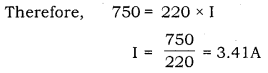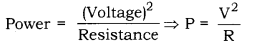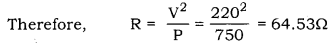# An electric iron has a rating of 750W, 220V. Calculate. (i) Current passing through it

An electric iron has a rating of 750W, 220V. Calculate.
(i) Current passing through it, and
(ii) Its resistance, when in use.

(i) Power = Voltage × Current
Let, the current passing through the bulb be ITherefore, 3.41 A current passes through the electric iron.

(ii) Electric power is related to voltage and resistance as:It is given that, the rating of the electric bulb is 750W, 220V.Therefore, the electric iron offers 64.53Ω resistance when in use.